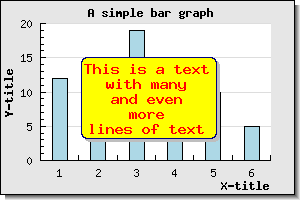## 14.17. Adding arbitrary texts to the graph

In much the same was as icon images can be added to the plot so can arbitrary text strings be added. This is done by creating an or several instances of the Text class (one per string that is needed). This feature is typically used to add clarifications or other information related to the actual graph.

These text instances are then added to the graph via the normal `Graph::Add()` method.

The position of the text in the image/graph can be given as either absolute pixels, fractions of the width/height of the graph or as scale values according to the specified (or automatically determined) scale.

To show some ways of positioning the text we use a very simple bar graph not to distract from the text. We first just add a single text line with most of the settings there default value. We do this by adding te following lines

 ```1 2 3 4 5 6 7 8 9 10 11 12 13 14 15 ``` ```SetPos(0, 20); // Set color and fonr for the text \$txt->SetColor('red'); \$txt->SetFont(FF_FONT2,FS_BOLD); // ... and add the text to the graph \$graph->AddText(\$txt); ?>```

Teh resulting graph can be seen in Figure 14.96

Figure 14.96. Adding a text object to a graph `(example25.php)`Let's make the text stand out a bit more by having a background color, framing the text box and adding a drop shadow by using the method `Text::SetBox()` The resulting graph can be seen in Figure 14.97

Figure 14.97. Making the text stand out a bit more by adding a background color and frame `(example25.1.php)`In order to use a text with several lines each line needs to be separated by a newline ("\n'"character). The default paragraph alignment is left edge but as was discussed in ?? paragraph alignment can be adjusted.

In our final example we a text with several line are place in the middle of the plot area.

Figure 14.98. Adding a text object with multiple rows of text. Paragraph alignment is set to "center" `(example25.2.php)`### Tip

To set the position of the text using scale positions instead use the method

• Text::SetScalePos(\$aX,\$aY)